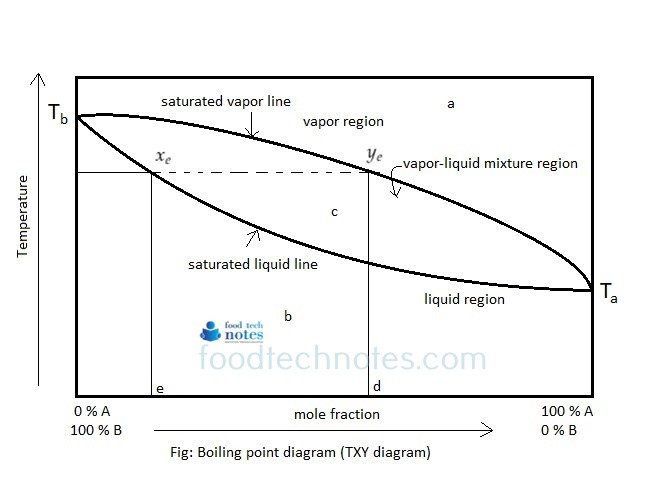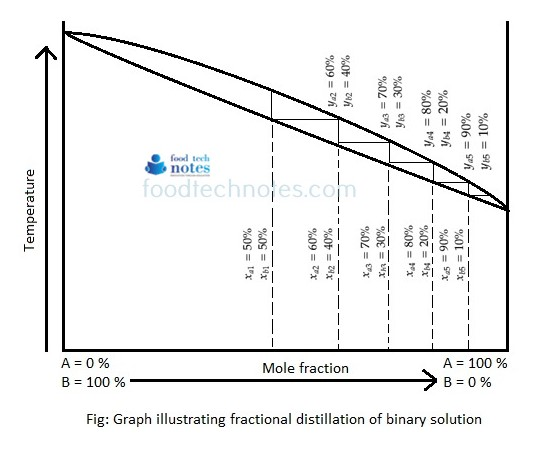# Boiling Point Diagram (TXY Diagram)

Equilibrium diagram (X-Y) diagram is simple diagram than boiling point diagram in which plot of temperature is eliminated. The boiling point diagram represents dynamic equilibrium of mole fraction of vapor and liquid phase at certain temperature at constant pressure. Boiling point diagram at constant pressure for binary mixture having component A and B is as below.In above figure shows binary mixture Ta represents boiling point of component A and Tb represents boiling point of component B. since Ta < Tb, component A is more volatile. In figure, abscissa contains more fraction or concentration and ordinate contains temperature. The diagram contains a curve whose ends coincides.

At any point, such as point ‘Ye’ on the upper curve represents vapor that will just begin to condense at temperature T and will give the liquid of the concentration (mole fraction) ‘d’. The lower point curve is called boiling point curve.

Any point on horizontal straight line such as Xe and Ye represents mole fraction of liquid and vapor in equilibrium at temperature given by their coordinates.

At point above the top line, such as point ‘a’ a mixture will be completely in vapor phase. All point below bottom line such as point ‘b’ the mixture is completely liquid. Point between two curves such as point ‘c’, system is partly liquid and partly vapor.

Suppose the liquid mixture of composition ‘x’ is heated slowly, it begins to boil at temperature T. The first vapor will have a definite composition represented on diagram by ‘Ye’. As soon as appreciable amount of vapor has been formed, the composition of liquid will no longer corresponds to ‘d’. Since vapor is richer in more volatile component than liquid from which it was evolved and hence the concentration of liquid will no longer be there and tends to move to left on the liquid curve.

Another demonstration on how fractionation distillation works and its relative data plot in TXY diagram is shown below.